Matplotlib examples: Number Formatting for Axis Labels

Last updated:

Full code available on this jupyter notebook

Comma as thousands separator

Formatting labels must only be formatted after the call to plt.plot()!

Example for y-axis: Get the current labels with .get_yticks() and set the new ones with .set_yticklabels() (similar methods exist for X-axis too):

import matplotlib.pyplot as plt
import numpy as np

# generate sample data for this example
xs = [1,2,3,4,5,6,7,8,9,10,11,12]
ys=np.random.normal(loc=10000,size=12, scale=20000) + 100000

# plot the data
plt.bar(xs,ys)

# after plotting the data, format the labels
current_values = plt.gca().get_yticks()
plt.gca().set_yticklabels(['{:,.0f}'.format(x) for x in current_values])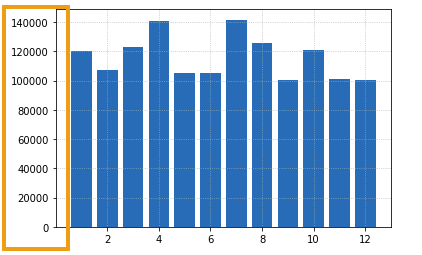BEFORE: no formatting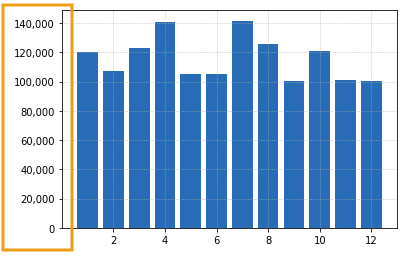AFTER: thousands separated with commas

Disable scientific notation

As before, labels must only be formatted after the call to plt.plot()!

Example for y-axis: Get the current labels with .get_yticks() and set the new ones with .set_yticklabels() (similar methods exist for X-axis too)

import matplotlib.pyplot as plt
import numpy as np

# generate sample data for this example
xs = [1,2,3,4,5,6,7,8,9,10,11,12]
ys=np.random.normal(loc=0,size=12, scale=500000) + 1000000

# plot the data
plt.bar(xs,ys)

# after plotting the data, format the labels
current_values = plt.gca().get_yticks()
# using format string '{:.0f}' here but you can choose others
plt.gca().set_yticklabels(['{:.0f}'.format(x) for x in current_values])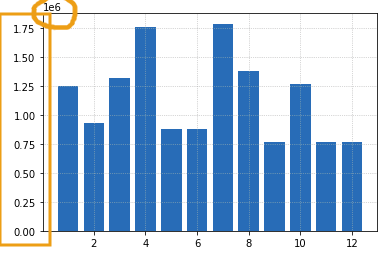BEFORE: matplotlib will sometimes add
scientific notation when numbers are large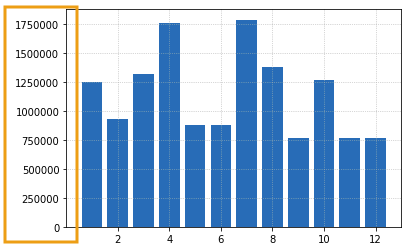AFTER: no scientific notation

Format y-axis as Percentages

similar methods exist for X-axis too

Once again, get current labels with .get_yticks(), set new labels with .set_yticklabels():

import matplotlib.pyplot as plt
import numpy as np

# generate sample data for this example
xs = [1,2,3,4,5,6,7,8,9,10,11,12]
ys= np.minimum(np.random.normal(loc=0.5,size=12,scale=0.4), np.repeat(1.0, 12))

# plot the data
plt.bar(xs,ys)

# after plotting the data, format the labels
current_values = plt.gca().get_yticks()
# using format string '{:.0f}' here but you can choose others
plt.gca().set_yticklabels(['{:,.0%}'.format(x) for x in current_values])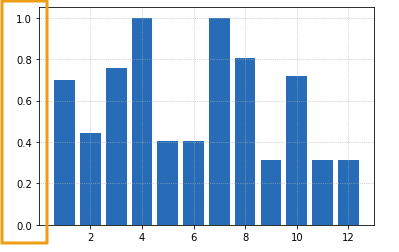BEFORE: y-axis with float numbers
that represent percentages or probabilitiesAFTER: numbers between 0 and 1 become percentages